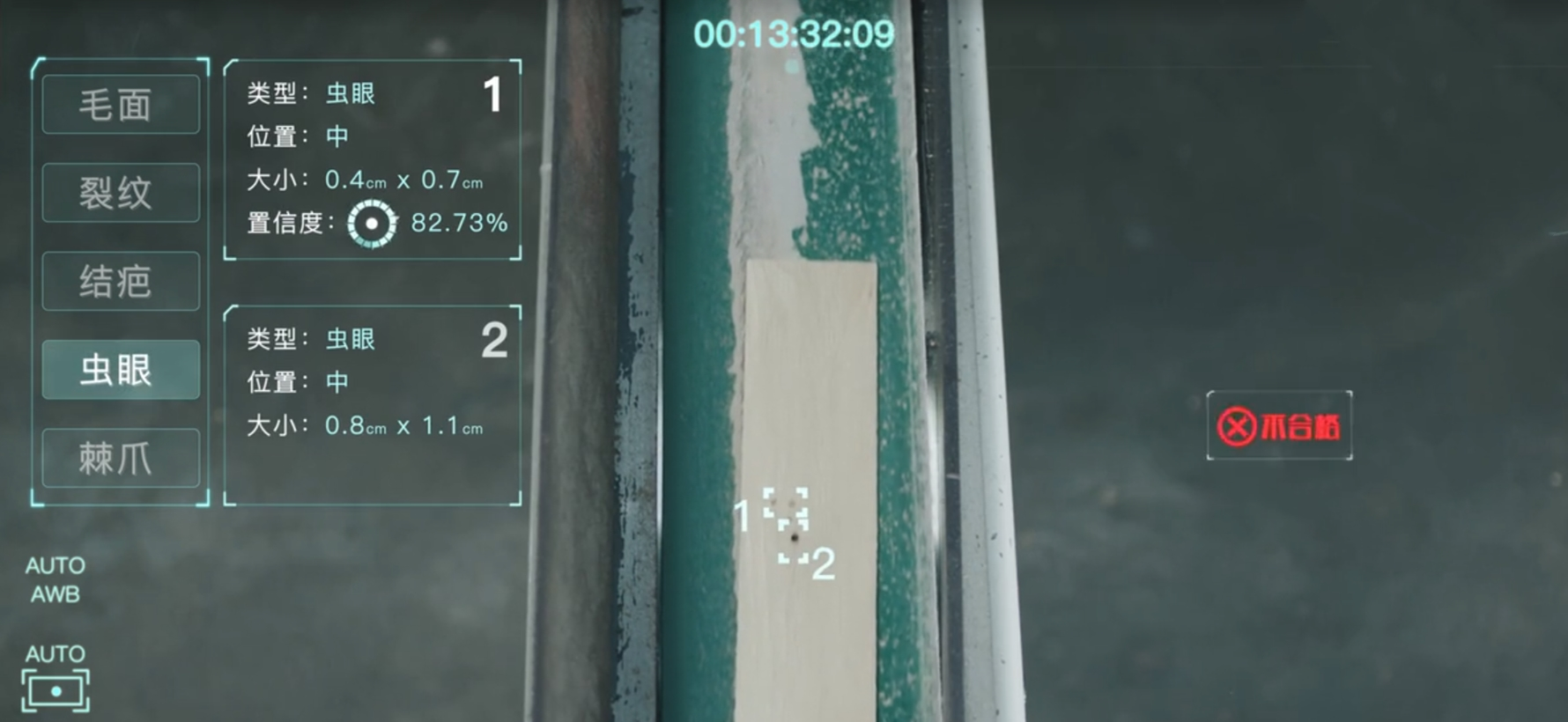# 学习新技能：Python and AI# 列表和字典

## 列表

students = [‘小明’,’小红’,’小刚’]
print (students)

students = [‘小明’,’小红’,’小刚’]
print(students[:2])

### append()函数

append()函数可以给列表增加元素。用append()给列表增加元素，每次只能增加一个元素（可以是字符串、数字，或列表本身）。其并不生成一个新列表，而是让列表末尾新增一个元素。而且，列表长度可变，理论容量无限，所以支持任意的嵌套。例如：

students = [‘小明’,’小红’,’小刚’]
students.append(‘小美’)
print (students)

### del语句

students = [‘小明’,’小红’,’小刚’,’小美’]
del students
print (students)

# 字典

```students = ['小明','小红','小刚']
scores = {'小明':95,'小红':90,'小刚':90}```

scores = {‘小明’:95,’小红’:90,’小刚’:90}
print (scores[‘小红’])

scores = {‘小明’:95,’小红’:90,’小刚’:90}
del scores[‘小刚’]
scores[‘小刚’] = ’92’
scores[‘小美’] = ’85’

print (scores)

```scores = {'小明':95,'小红':90,'小刚':90}
#del scores['小刚']
#如果只需要修改键里面的值，可不需要del语句
scores['小刚'] = 92
```

`students = [['小明','小红','小刚','小美'],['小强','小兰','小伟','小芳']]`

```students = [['小明','小红','小刚','小美'],['小强','小兰','小伟','小芳']]
print(students)
# 1是外层列表包含小芳的元素的偏移量，3是被嵌套的列表中小芳的偏移量。```

```scores = {
'第一组':{'小明':95,'小红':90,'小刚':100,'小美':85},
'第二组':{'小强':99,'小兰':89,'小伟':93,'小芳':88}
}
print(scores['第二组']['小芳'])```

```# 最外层是大括号，所以是字典嵌套列表，先找到字典的键对应的列表，再判断列表中要取出元素的偏移量
students = {
'第一组':['小明','小红','小刚','小美'],
'第二组':['小强','小兰','小伟','小芳']
}
print(students['第一组'])
#取出'第一组'对应列表偏移量为3的元素，即'小美'

# 最外层是中括号，所以是列表嵌套字典，先判断字典是列表的第几个元素，再找出要取出的值相对应的键
scores = [
{'小明':95,'小红':90,'小刚':100,'小美':85},
{'小强':99,'小兰':89,'小伟':93,'小芳':88}
]
print(scores['小强'])
#先定位到列表偏移量为1的元素，即第二个字典，再取出字典里键为'小强'对应的值，即99。```

# 循环

## for…in…循环语句

for i in [1,2,3,4,5]:

print(i)

for i in range(3):
—-print(i)

for n in range(11):
—-print(‘书桓走的第’+ str(n) + ‘天，想他’)

range()函数还有一种用法，例如：

for i in range(0,10,3):
—-print(i)

## while循环

print (‘登陆成功’)

while循环，在满足条件的时候，会一轮又一轮地循环执行代码。注：缩进后的【while子句】才会被循环执行，例如：

```a = 0
while a < 5:
​    a = a + 1
print(a)

# 与下面的代码执行结果不同
a = 0

while a < 5:
​    a = a + 1
print(a)```

## for…in…循环和while循环的区别

for循环和whlie循环最大的区别在于【循环的工作量是否确定】，for循环就像空房间依次办理业务，直到把【所有工作做完】才下班。但while循环就像哨卡放行，【满足条件就一直工作】，直到不满足条件就关闭哨卡。当【工作量确定】的时候，可以让for循环来完成重复性工作。反之，【工作量不确定时】可以让while循环来工作。有一种情况for循环和while循环都可以解决问题，那就是【把一件事情做N遍】。